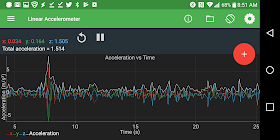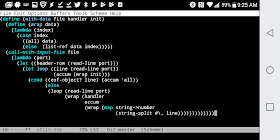## Thursday, November 09, 2017

### Calculating Distance from a Cell Phone's Accelerometer - A failed, but fun attempt

Our gym had a nifty Bluetooth enabled jump rope that would report how many jumps you made in a session. Then the rope broke. This left me thinking: could I approximate the same thing by using the sensors on my phone?

Poking around, I learned that there's a class of app that gives you access to these sensors. In that genre, Physics Toolbox Suite appears to be a top pick. Launching the app gives me access to all sorts of data, from the gyroscope, to barometric pressure, to the GPS. It also gives me a way to record the data for a period of time, generating a simple CSV file. And if all that weren't enough, it's also ad free. To show my support, I just purchased the Pro version, even though the standard version does everything I need.

What caught my eye in the app was the Linear Accelerometer screen:I've long since forgotten the majority of high school physics lessons, but I do know this: units matter. As the app reminded me (or perhaps taught me), acceleration is measured in meters per second, squared. What jumped out at me was the presence of meters, or distance. I now had my goal: rather than count the number of jumps in a jump-rope session, wouldn't it be far cooler to count distance? That is, how many vertical meters did I manage to conquer while jumping rope. I wasn't sure how I was going to tease this data out of the accelerometer, but I knew I was going to try.

The first stop in my journey was to head over to YouTube and learn how acceleration and distance are 'connected.' I found this Khan Academy video gave me enough background on distance, velocity and acceleration to let me derive a solution. The Physics Toolbox Suite app would give me a CSV file that provide an acceleration value every 100th of a second. Thinking through these concepts, I realized that I could at each moment derive speed and then distance, by looking at the change in time and acceleration. And because I knew that I was starting by standing still (that is, my velocity was zero), the whole system could be initialized and in theory, a final answer derived. I was quite proud of myself. Shira, for her part, rattled off the exact formula and explained to me that this super basic physics. Apparently, I could have skipped the Kahn Accademy, and just talked to her.

Now that I had a method for calculating distance via the accelerometer, I needed to try it in a controlled environment. I laid down a tape measure, and noted 4 meters. I then walked these 4 meters while capturing the accelerometer data via the Physics Toolbox app. With a few test runs captured, it was time to do the fun part: program the above solution.

Because the data was on my phone, I thought it appropriate to code the solution on my phone. I busted out tinyscheme, emacs and termux. Tinyscheme is a delightful tool to work with because it's so lean. This can also be a source of frustration, as to implement the above solution I needed a way of reading form a CSV file, and I'd rather not have to implement the string and file handling. Fortunately, I was able to borrow some code from the Programming Praxis Standard Prelude (thanks!), which meant that I didn't have to implement string-split or read-line.

The following function does the heavy lift for reading and parsing the CSV file:

```;; Call handler on each row in the file.
;; Assume the first row is a header and the
;; following rows are all numeric data
(define (with-data file handler init)
(define (wrap data)
(lambda (index)
(case index
((all) data)
(else  (list-ref data index)))))
(call-with-input-file file
(lambda (port)
(let loop ((line (read-line port))
(accum (wrap init)))
(cond ((eof-object? line) (accum 'all))
(else
(wrap (handler
accum
(wrap (map string->number
(string-split #\, line)))))))))))))
```

Ah, it's such a join programming this sort of thing in Scheme. It's like programming with Play-doh: you can invent new constructs with so little effort.

With the file parsing out of the way, it was time to write the actual code to calculate distance:

```;; Use Physics 101 to calculate distance
;; based on the current acceleration, time
;; and velocity
(define (calculate-distance accum data)
(let* ((t-now (data 0))
(a-now (+
(data 1)  ; ax
(data 2)  ; ay
(data 3)  ; az
))
(t-prev (accum 0))
(v-prev (accum 1))
(d-prev (accum 2))
(t (- t-now t-prev))
(v-now (+ v-prev (* a-now t)))
(d-now (+ d-prev (* v-now t))))
(list t-now v-now d-now)))
```

There's nothing particular sexy here. I'm taking in the time and acceleration data (via data) and using my accumulated values to calculate the current velocity and distance.

It was now time for the moment of truth. I ran the code against a sample data:And, it was a bust. My code tells me that I covered 2.54 meters instead of the 4 meters I knew I covered.

I've been through the code a number of times, and I think the algorithm is fine. My guess is that I'm expecting too much accuracy out of my phone's sensor. Though, maybe my algorithm is fine and I just made a boneheaded mistake. You can see the full source code of the project here, and the data file is here.

Still, this exercise has been valuable. I learned about the Physics Toolbox app, which is definitely a keeper. I once again saw the amazing power of Termux, emacs, tinyscheme and how effortlessly they all come together on my cell phone. And I even learned some physics along the way.

While I think my goal of measuring how 'far' I jumped may not work. I'm still not convinced I can't use the sensors on my phone to at least count jumps. The data is there, the function to process the data file is ready, I just need to plug in right algorithm to make this all make sense.

Do you see where my assumptions on the accelerometer all fell apart? Please explain the comments!1.Um, Ben, you have just rediscovered that doing double integration makes your errors and noise accumulate at a quadratic rate ... There is a reason why nobody tries to measure distance using accelerometers using this method. It works, but the data will get unusable very quickly.

You can rely on it for a few seconds at best, then you will need to correct the distance estimate from some other source - this is what e.g. the Lighthouse tracking system in HTC Vive does. They integrate the accelerometer data 100+ times per second and then once in a while (several times per second) correct the drift using the laser based system.

If you want to use only an accelerometer, at best you can try to count steps - with each step manifesting itself as a "bump" in the accelerometer data. That will give you a rough estimate of distance traveled if you know the average length of your step. Many fitness applications do exactly this.

2.Thanks Jan!

I appreciate the detailed explanation. I had a feeling my method was too good to be true :-).

I'll have to give the 'bump' method a try next.

Thanks!